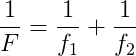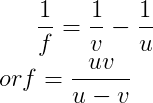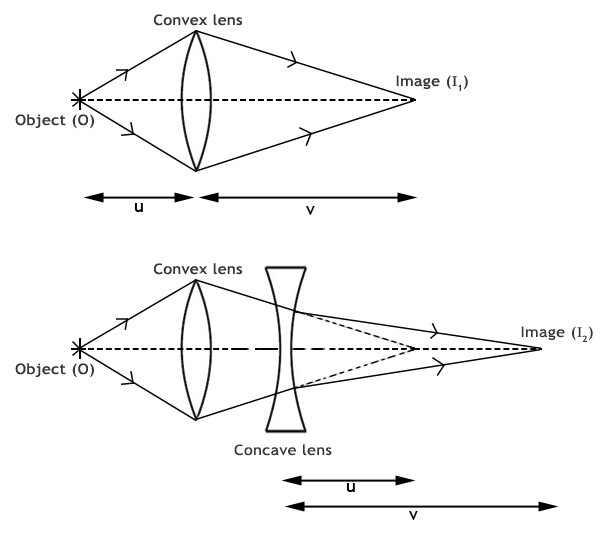Concave Lens - Focal Length

# Our Objective:

To find the focal length of a concave lens using a convex lens by:

• Lens in contact method
• Lens out of contact method

# The Theory:

## Concave lens

A concave lens is thinner at the center that at the edges. So the light beams passing through the lens are spread out or diverged. Therefore, the concave lens is called a diverging lens. The image formed by a concave lens is virtual and diminished.
Since a concave lens will not produce a real image, a convex lens is used to measure its focal length. There are two methods to find the focal length of a concave lens.

### a) Lens in contact method

When a concave lens of focal length f2 is placed coaxially in contact with a convex lens of focal length f1 and if F is the focal length of the combination, then,Therefore, the focal length of the concave lens,### b) Lens out of contact method

The real image (I1) formed by the convex lens will act as the virtual object for the concave lens. When concave lens is interposed between the convex lens and the real image I1, the new real image is formed at I2. If u is the distance of the concave lens from the virtual object I1 and v is the distance of the concave lens from the real image I2, then the focal length of the given concave lens is,# Learning outcomes:

• Students list the properties of concave lens.
• Students deduce and apply the lens formula.
• Students identify the two methods used to determine the focal length of concave lens.